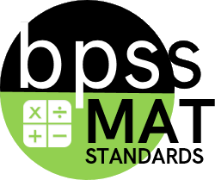#### MAT-04.NF.034th Grade (MAT) Targeted Standard
(NF) Number and Operations - Fractions
Cluster: Build fractions from unit fractions by applying and extending previous understandings of operations on whole numbers.

##### MAT-04.NF.03 Understand a fraction a/b with a > 1 as a sum of unit fractions 1/b.
a. Understand addition and subtraction of fractions as joining and separating parts referring to the same whole.
b. Decompose a fraction into a sum of fractions with the same denominator in more than one way, recording each decomposition with an equation. Justify decompositions by using a visual fraction model or other strategies.
c. Add and subtract mixed numbers with like-denominators.
d. Using visual fraction models and equations, solve word problems involving addition and subtraction of fractions referring to the same whole and having like denominators.